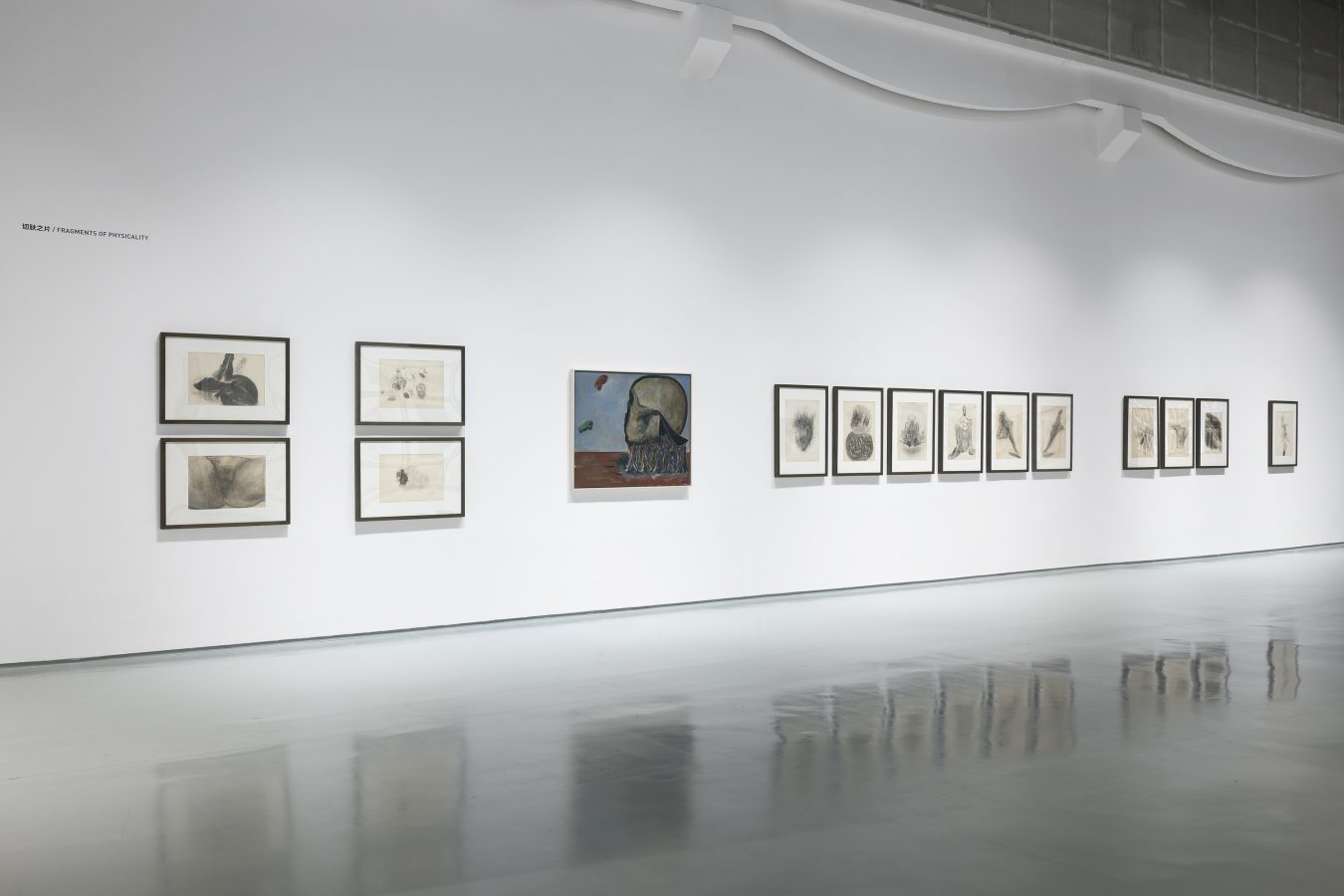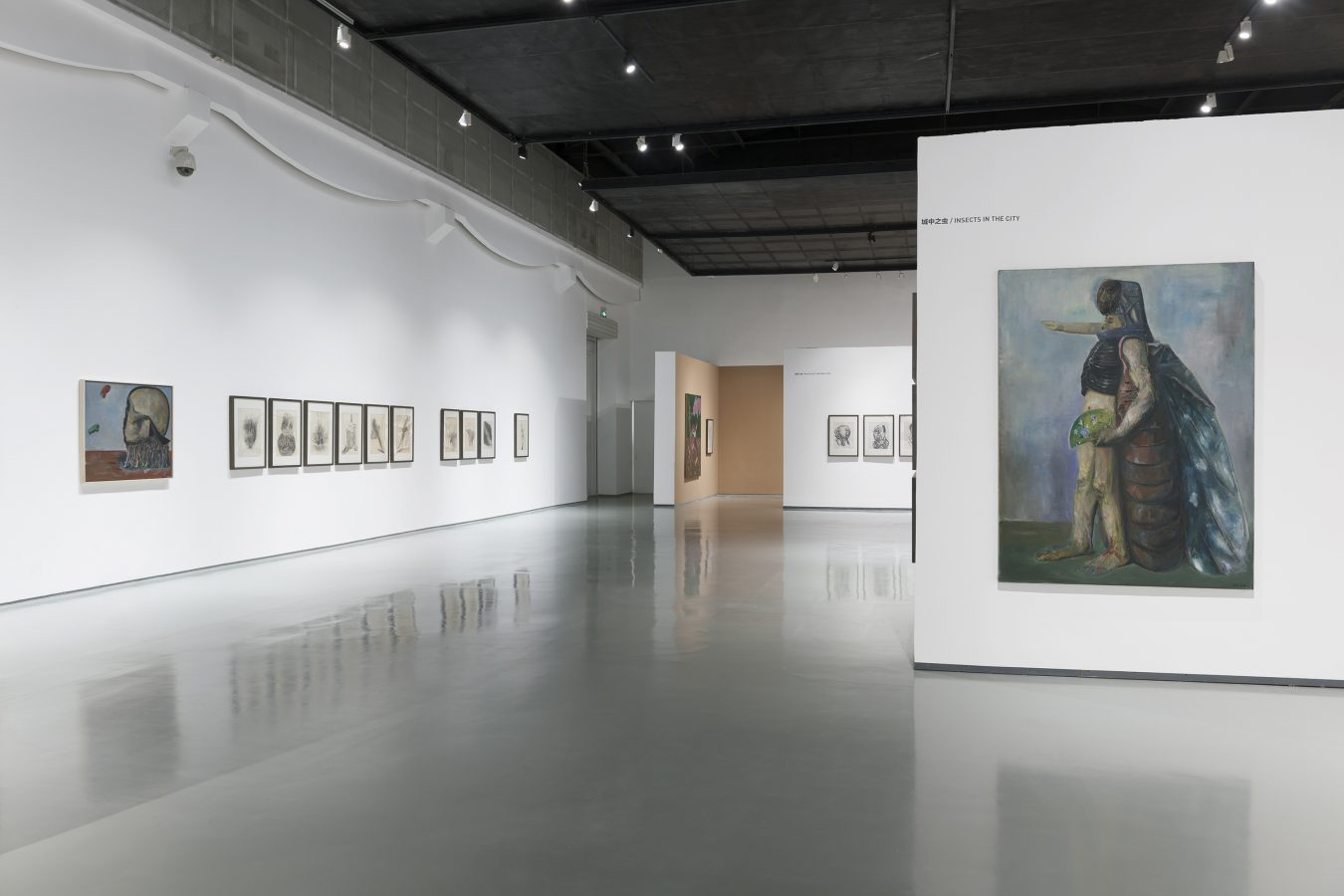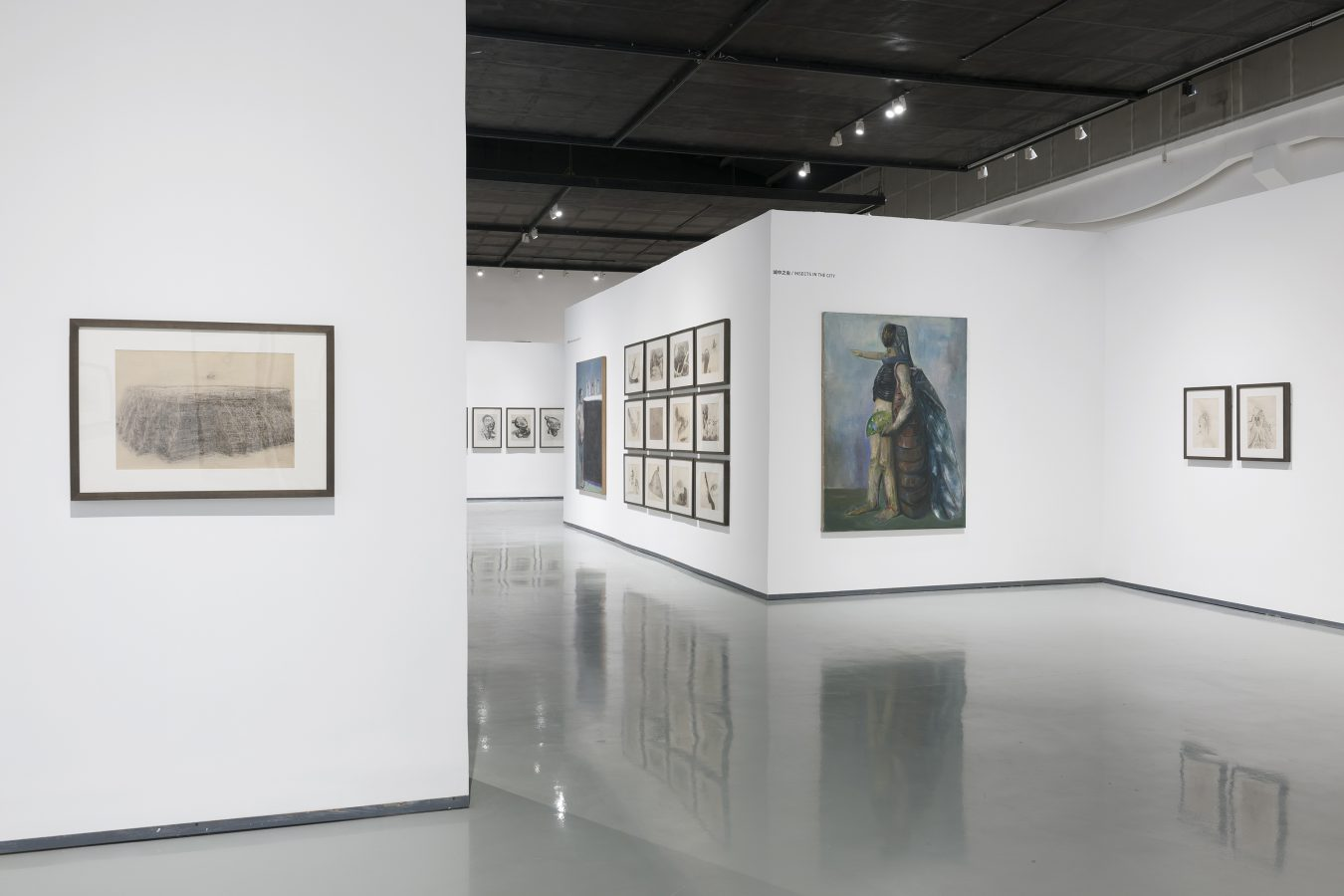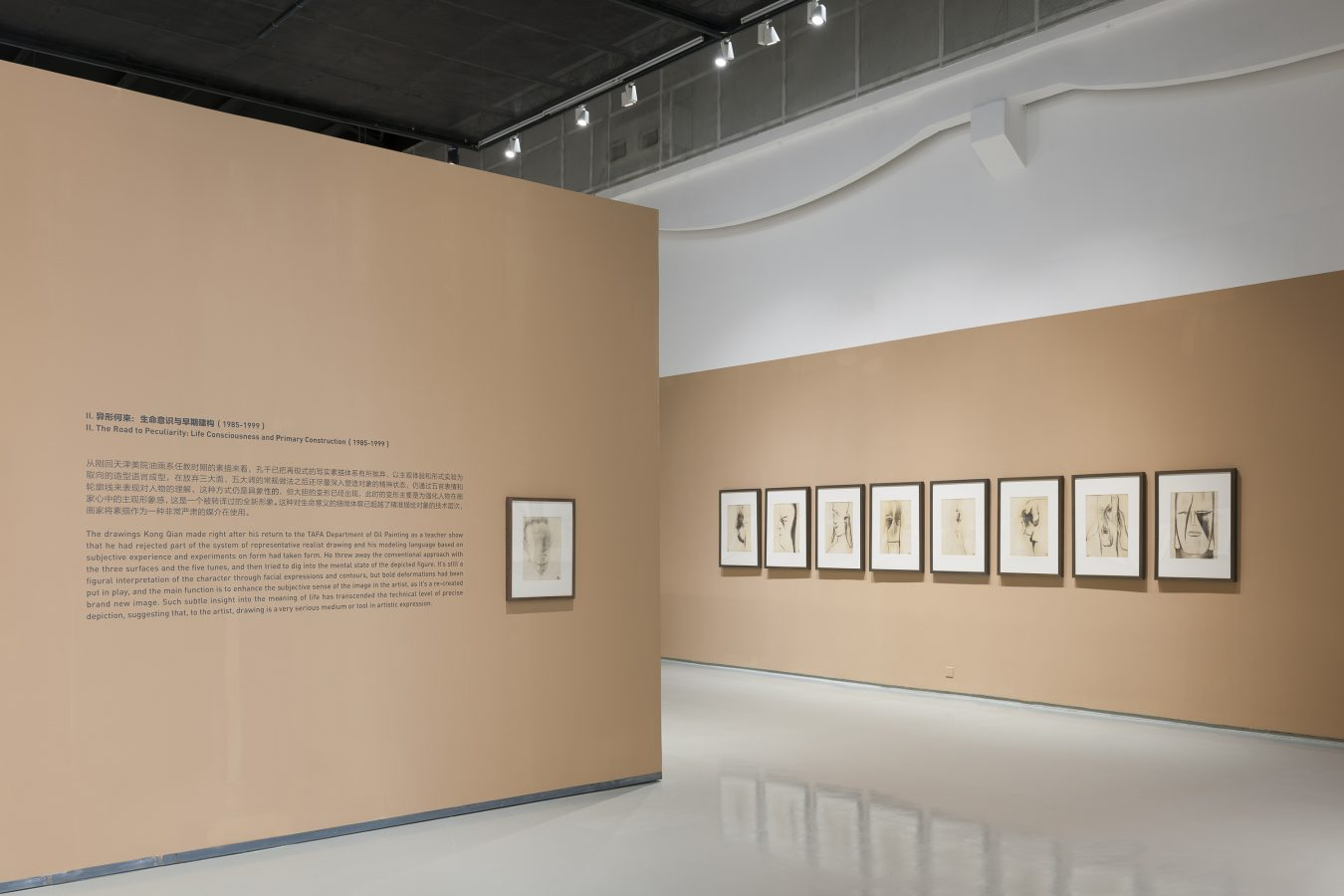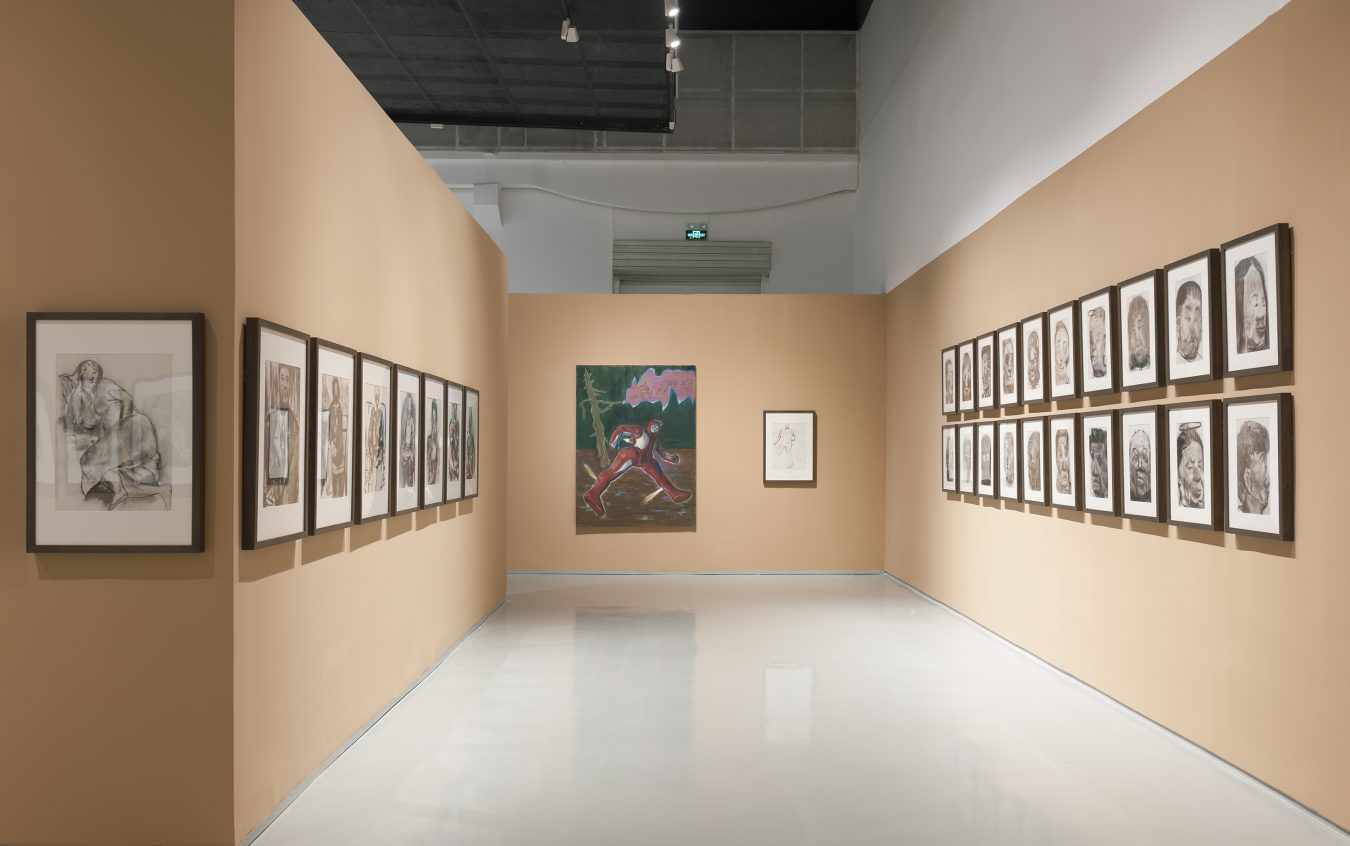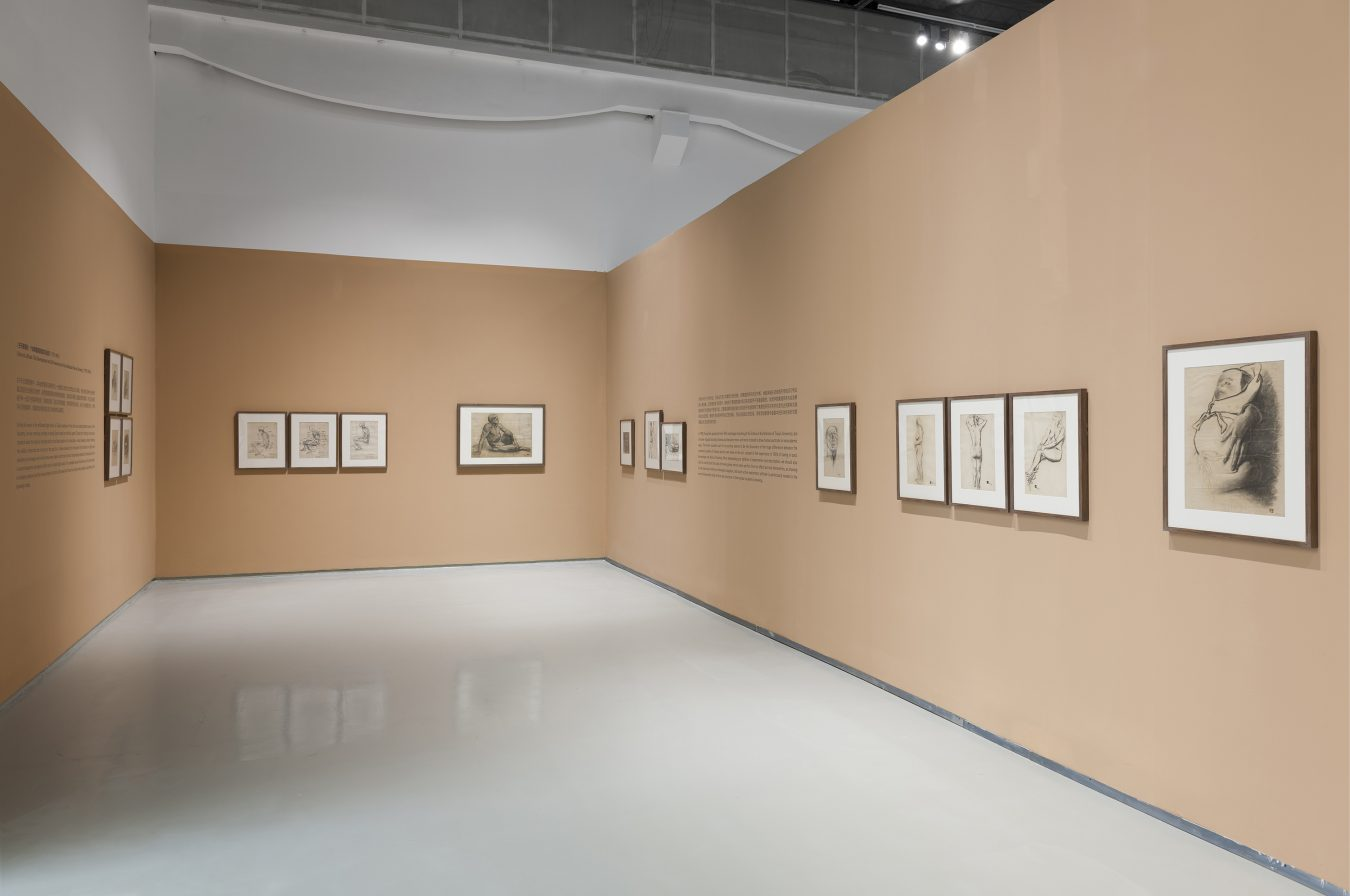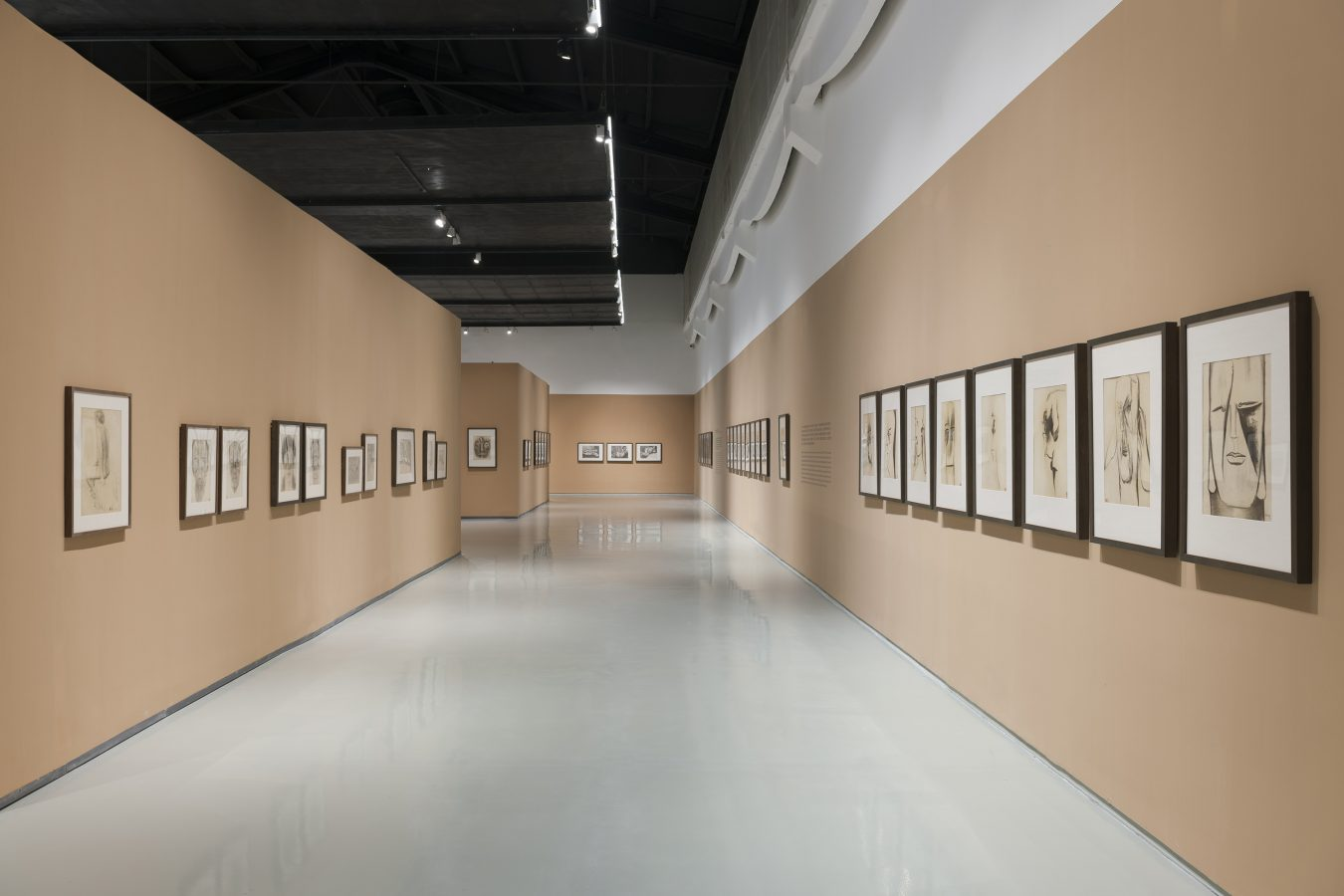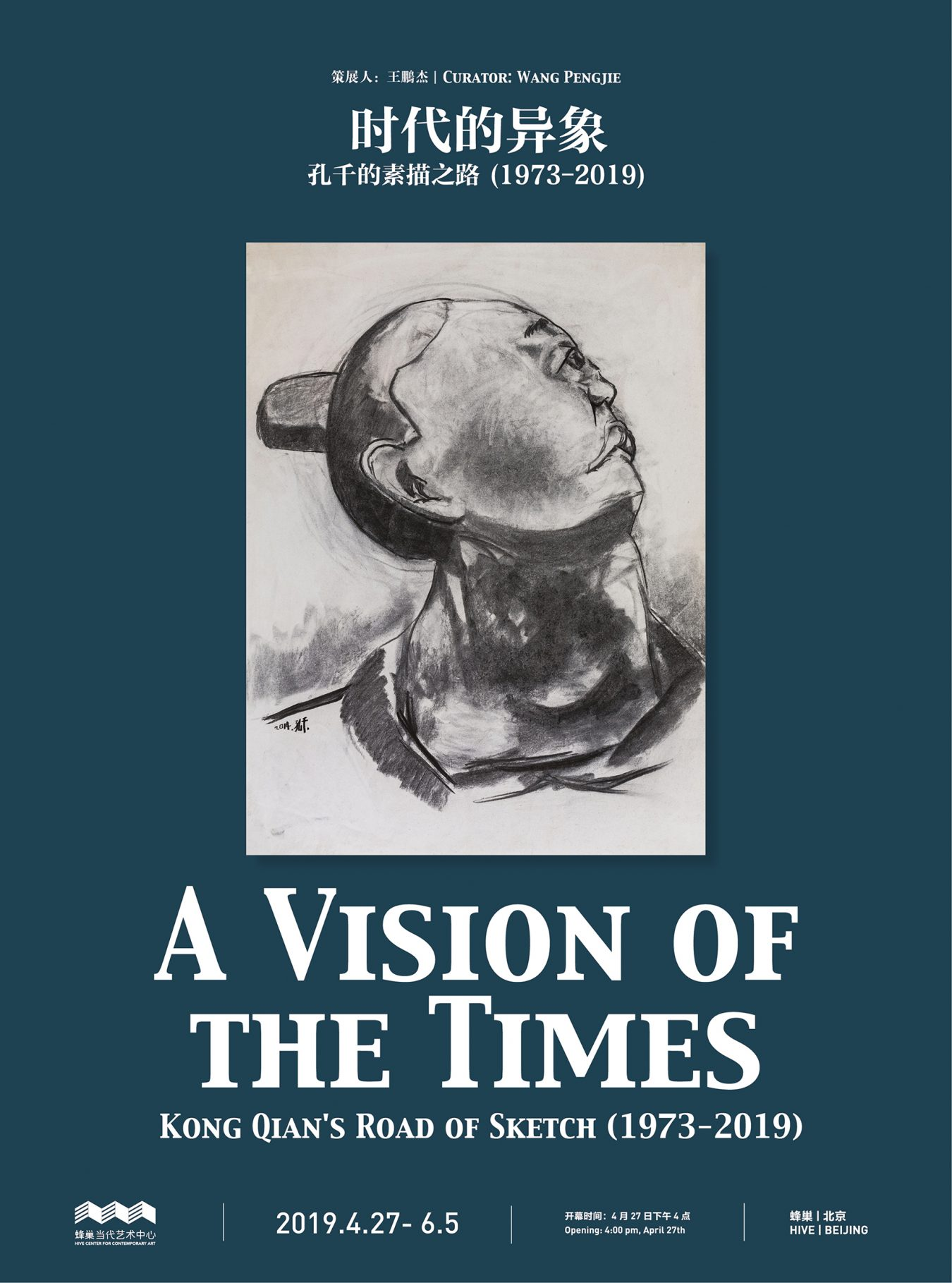2008. 布面油画. 193×155cm.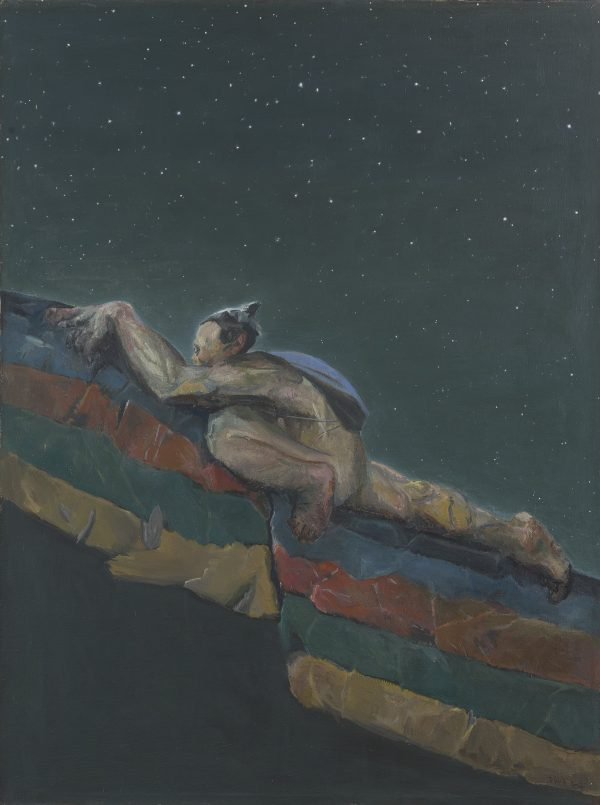2007. 布面油画. 200×150cm.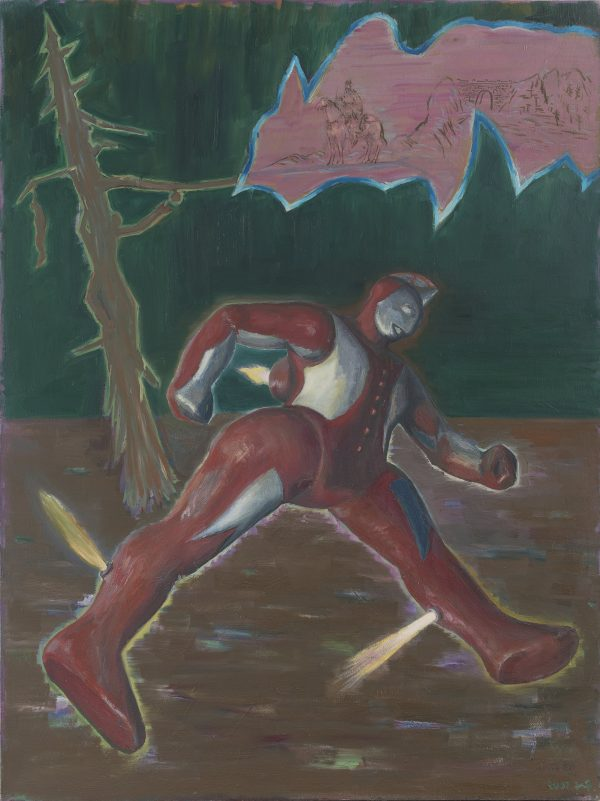2007. 布面油画. 200×150cm.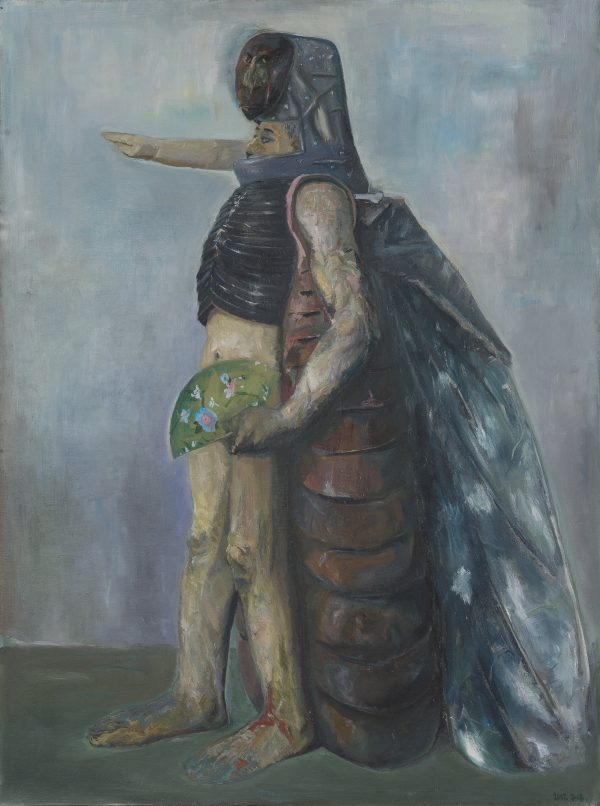2007. 布面油画. 200×150cm.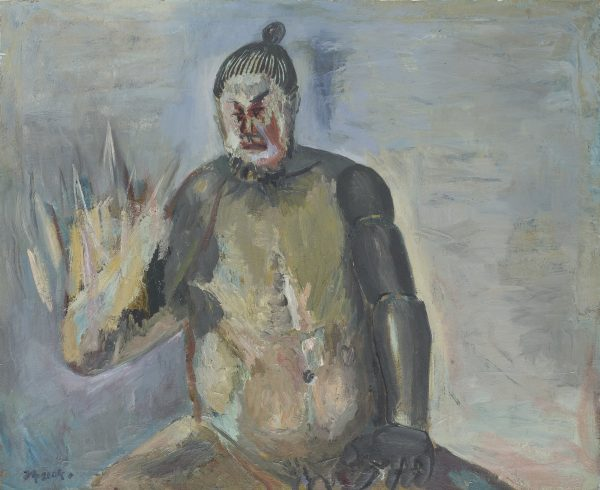2004. 布面油画. 90×110cm.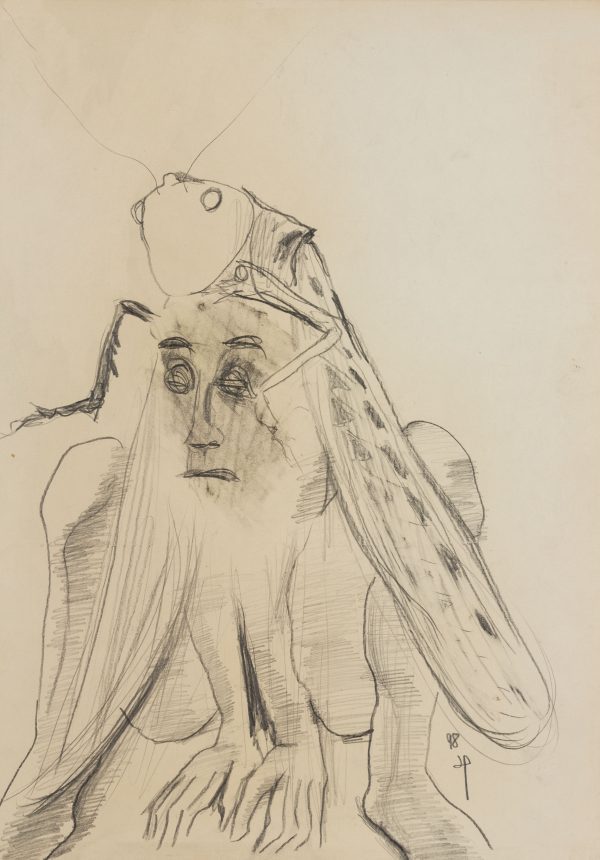1998. 纸本素描. 44×30cm.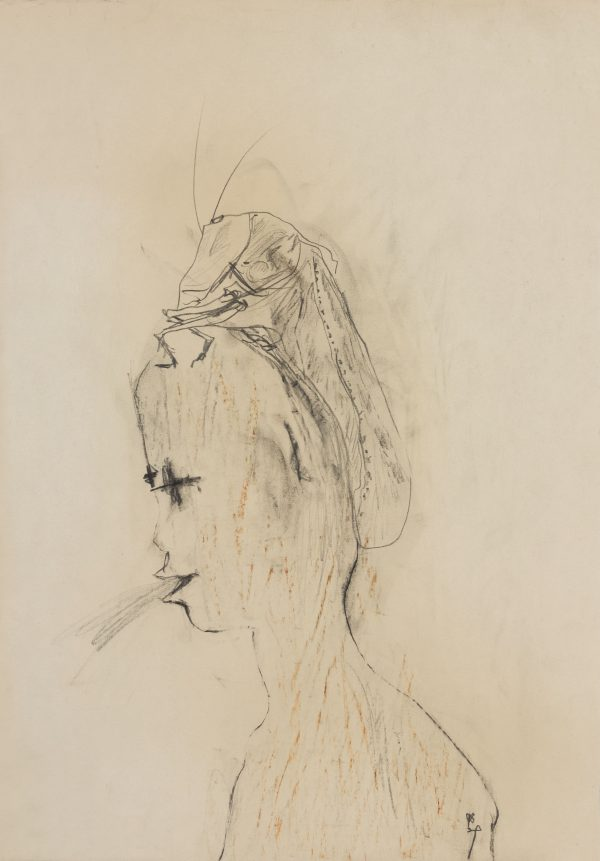1998. 纸本素描. 44.3×31cm.1998. 纸本素描. 54.5×39cm.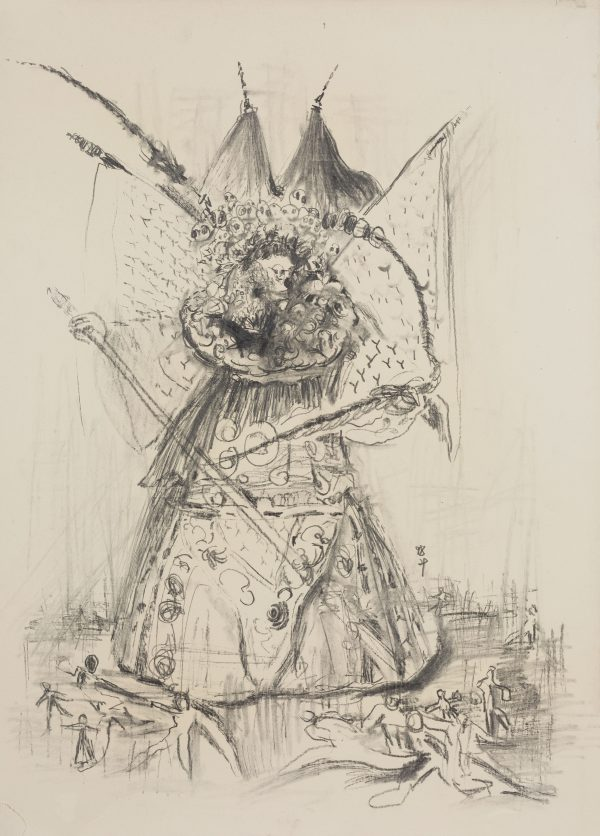1998. 纸本素描. 54.4×39.2cm.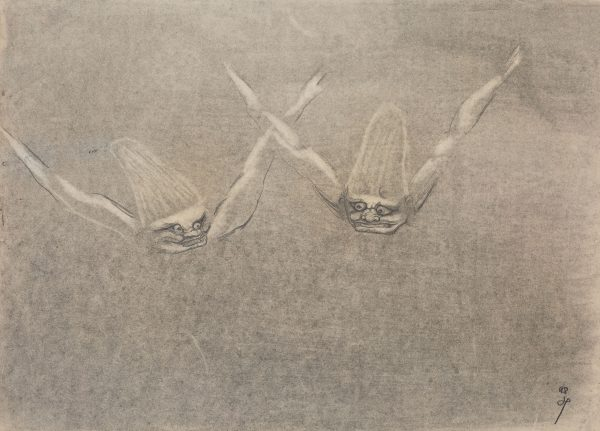Kong Qian

1998. 纸本素描. 39.2×54.3cm.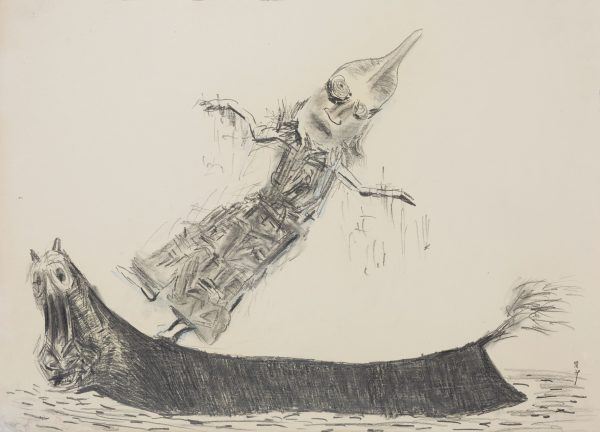1998. 纸本素描. 39.1×54.5cm.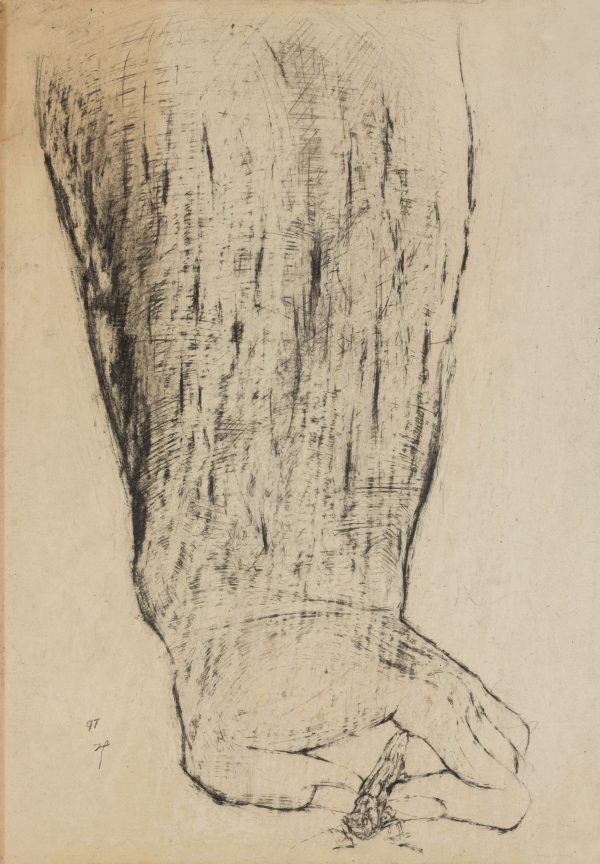1997. 纸本素描. 44.1×30.9cm.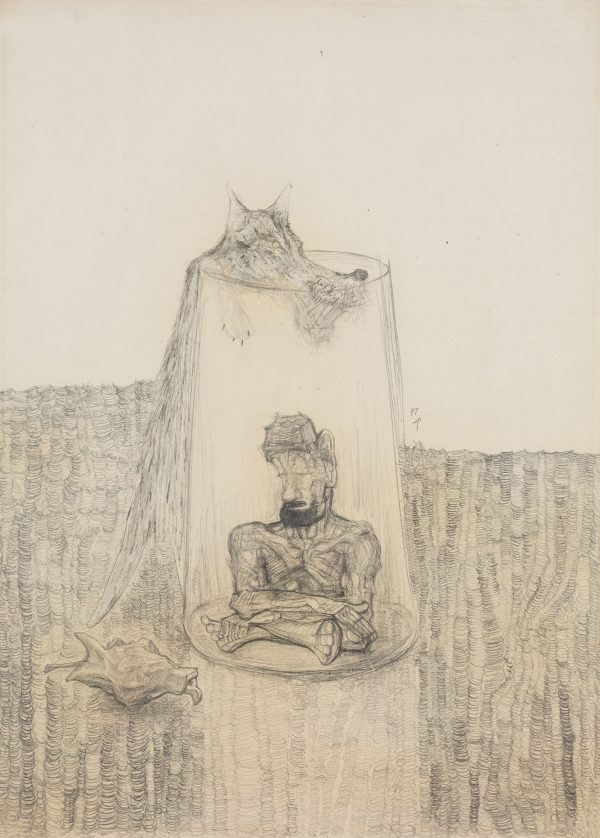1997. 纸本素描. 61.6×44.1cm.1997. 纸本素描. 44.3×30.9cm.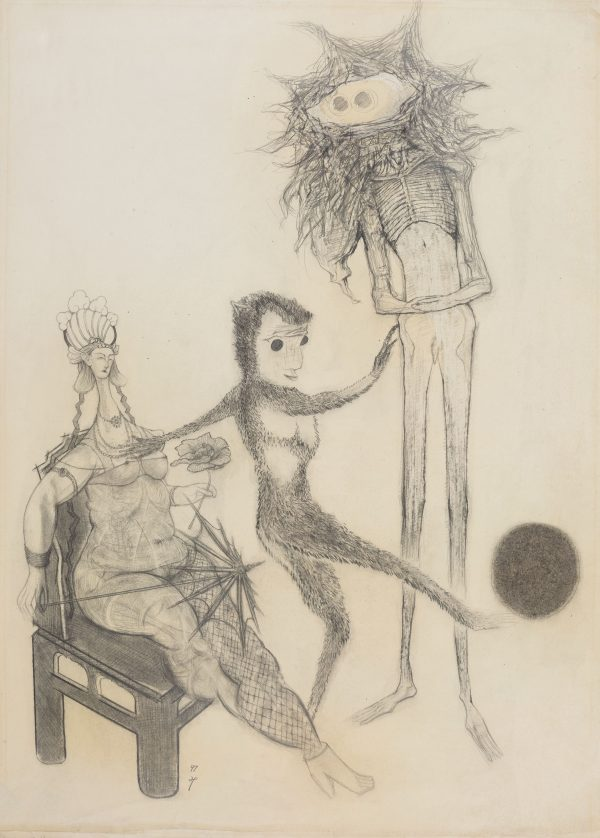1997. 纸本素描. 61.5×44cm.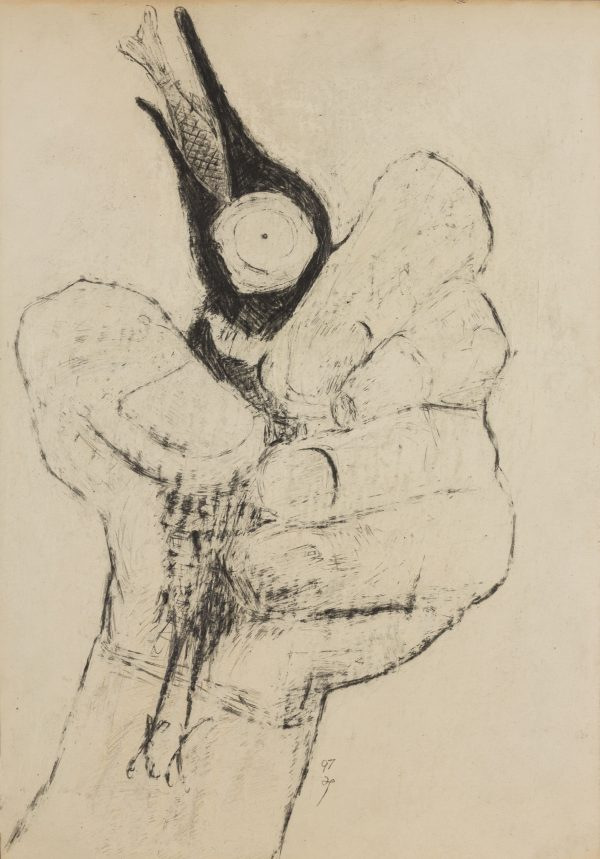1997. 纸本素描. 44.3×30.9cm.Kong Qian

1997. 纸本素描. 44.3×30.7cm.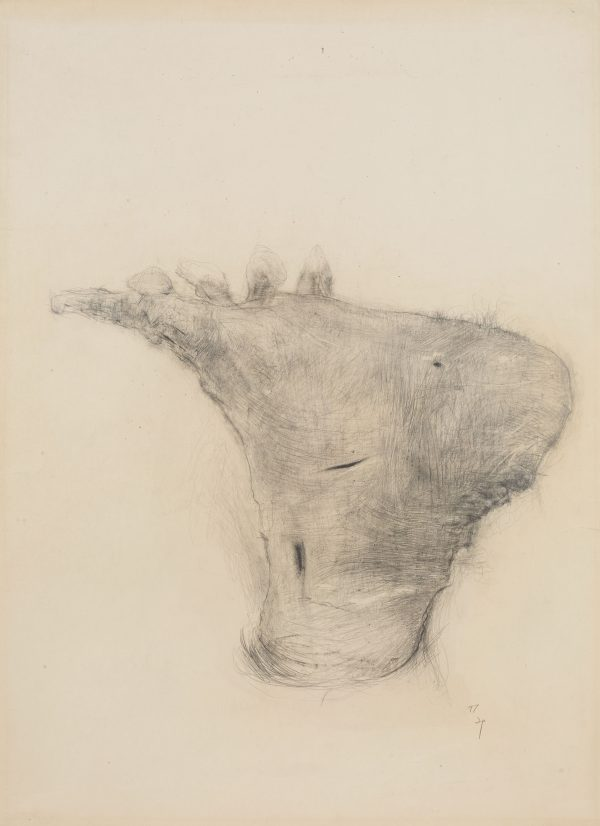1997. 纸本素描. 61.6×44.1cm.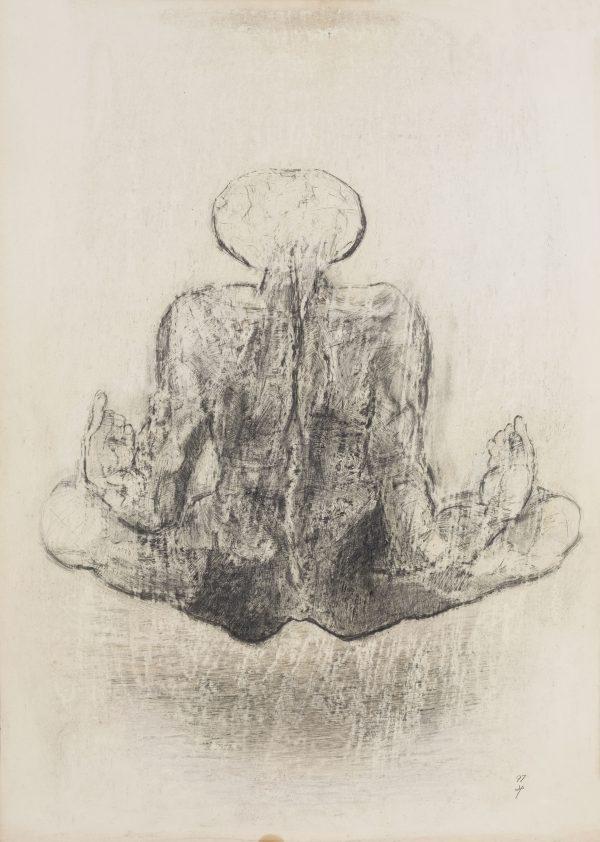1997. 纸本素描. 61.6×44.3cm.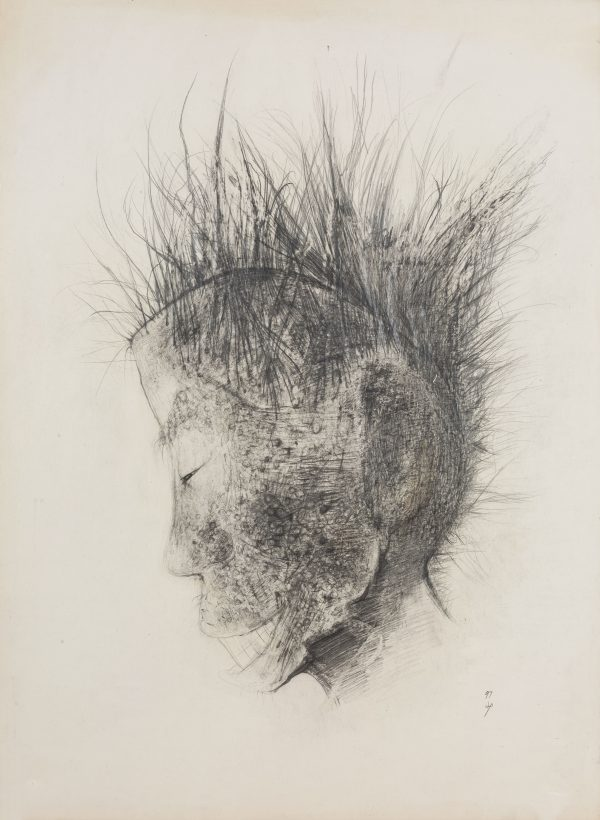1997. 纸本素描. 61.5×44.2cm.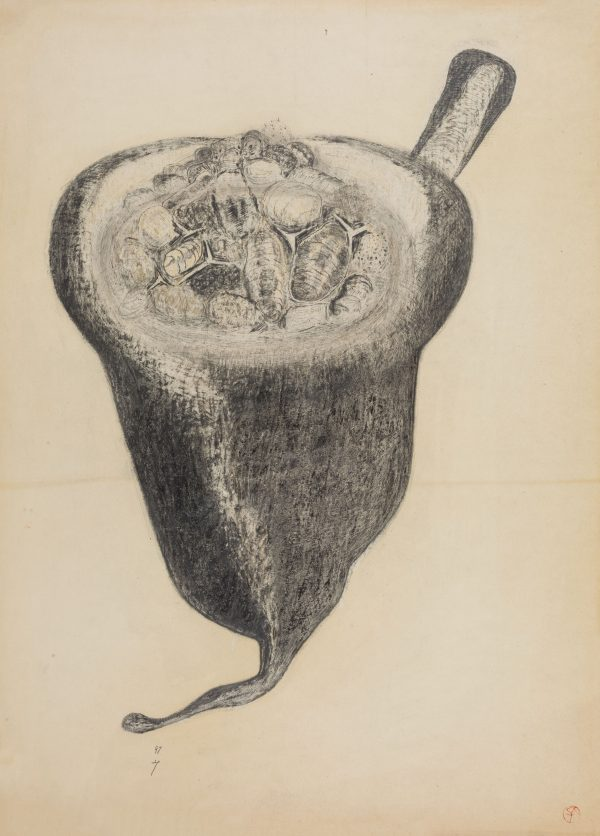1997. 纸本素描. 61.2×44.3cm.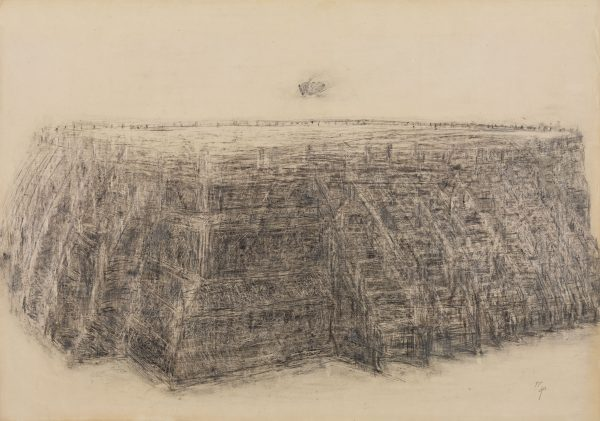1997. 纸本素描. 44.2×61.6cm.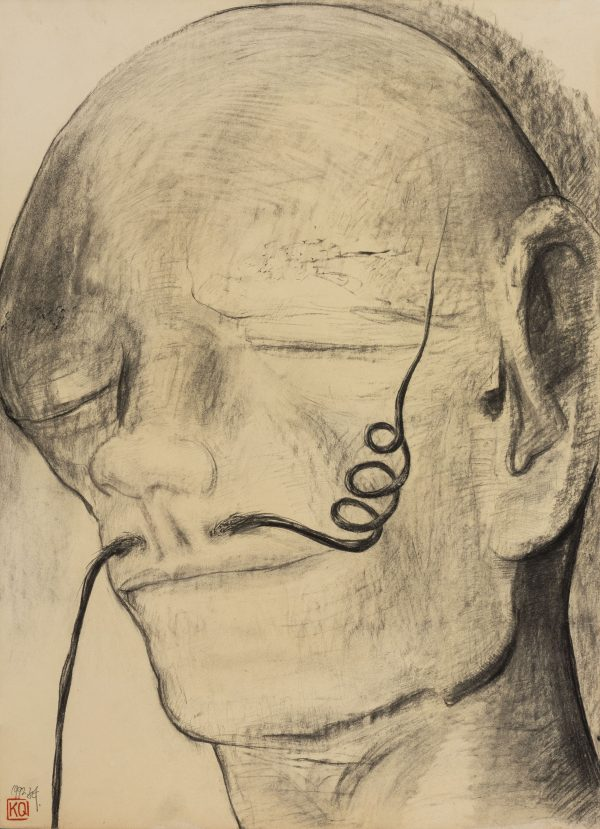1992. 纸本素描. 54.5×39cm.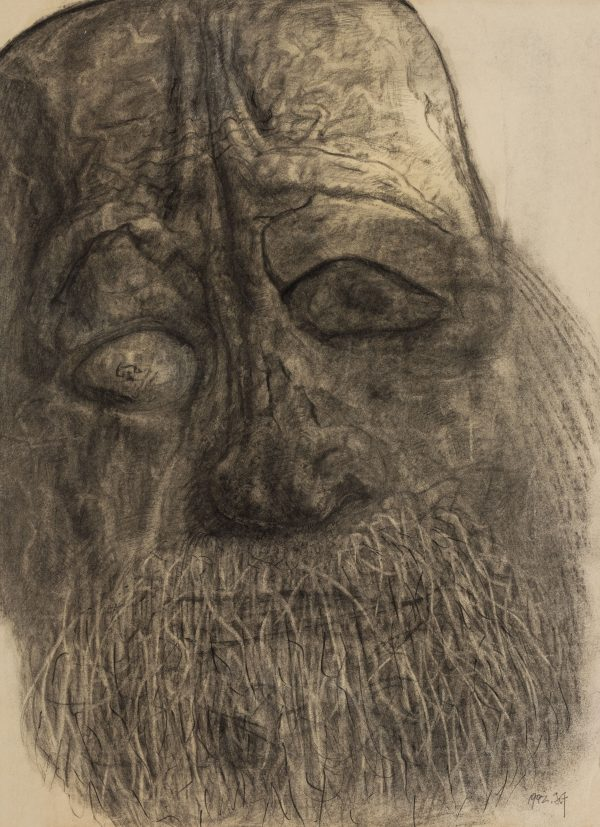1992. 纸本素描. 54.5×39cm.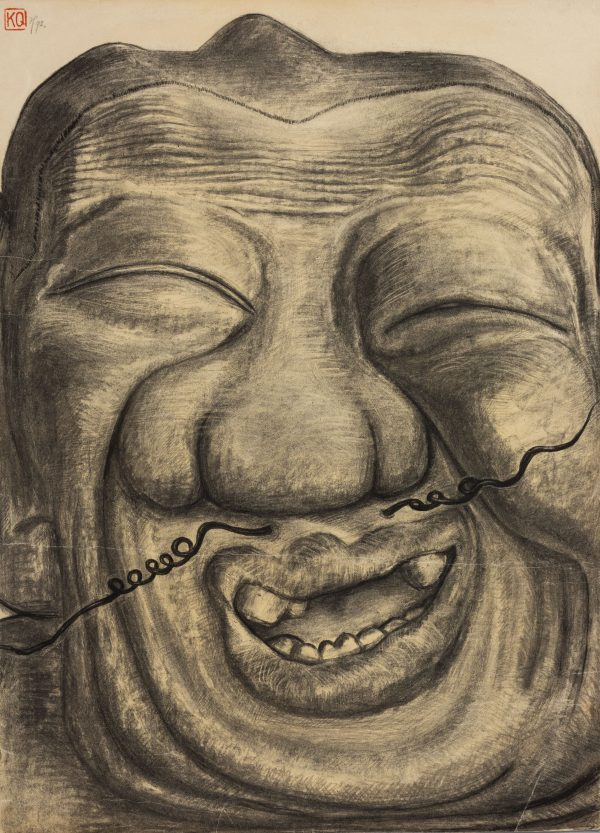1992. 纸本素描. 54.5×39cm.1992. 纸本素描. 39×27cm.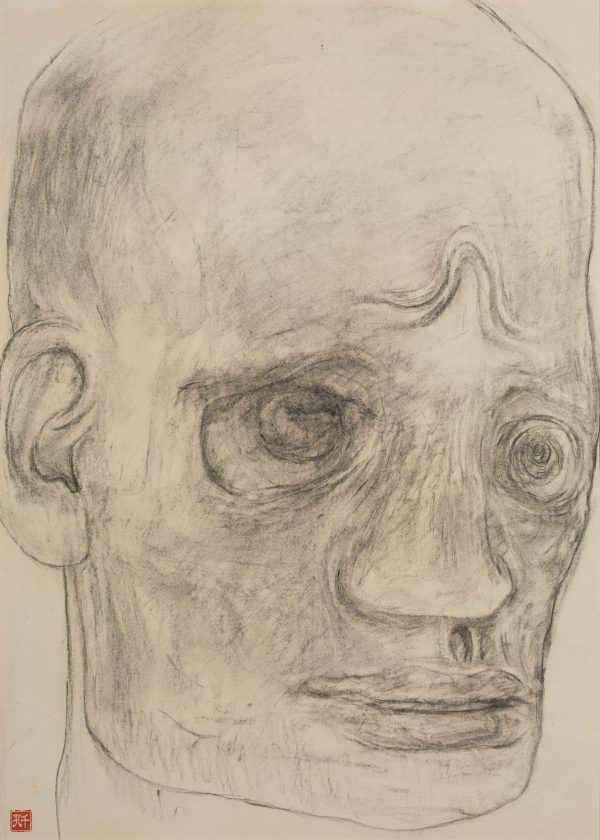1990. 纸本素描. 54.5×39cm.1990. 纸本素描. 54.5×39cm.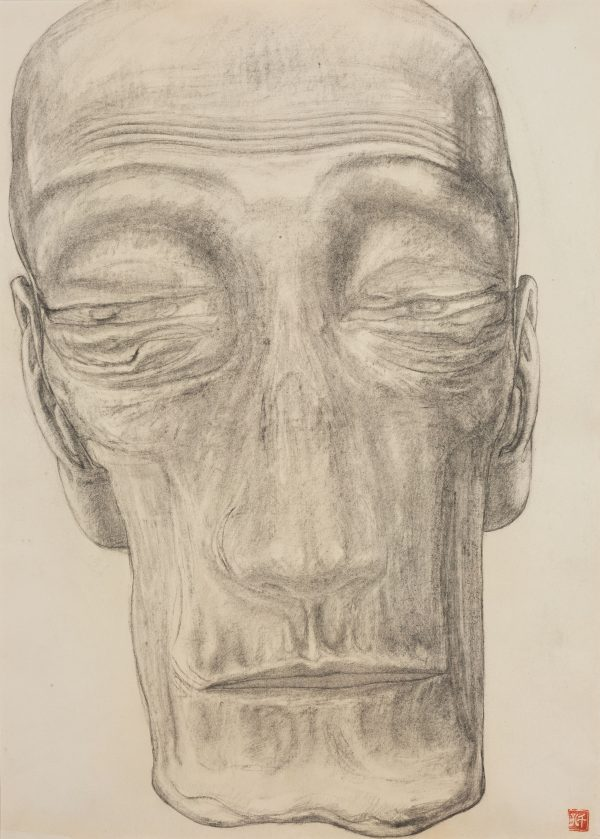1990. 纸本素描. 54.5×39cm.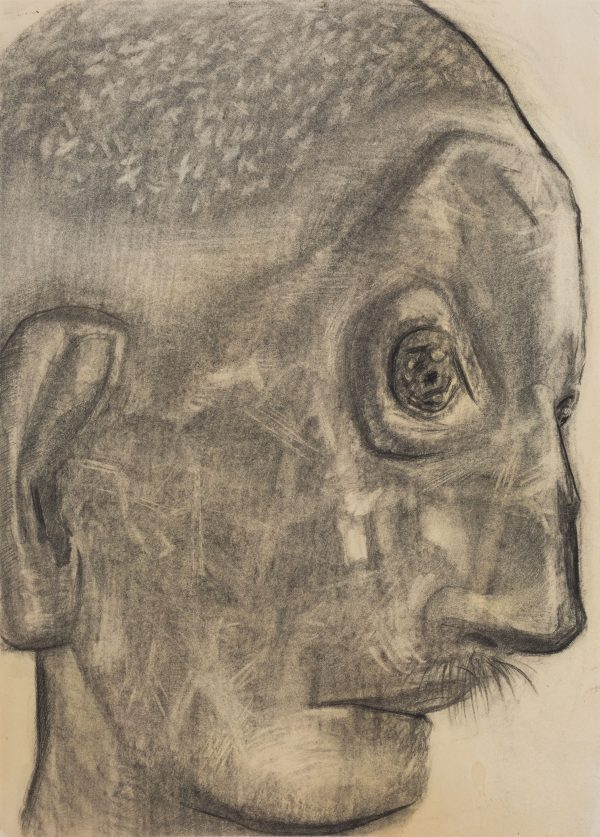1990. 纸本素描. 54.5×39cm.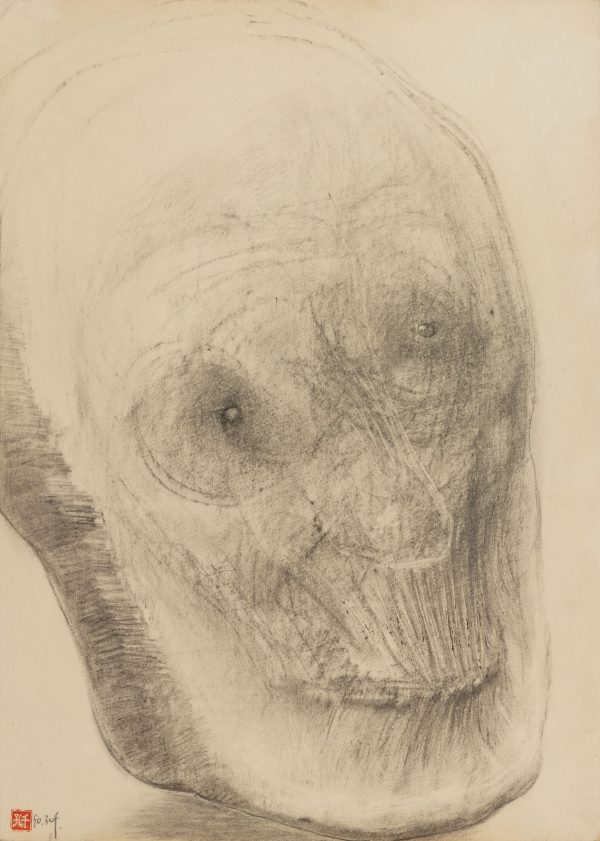1990. 纸本素描. 54.5×39cm.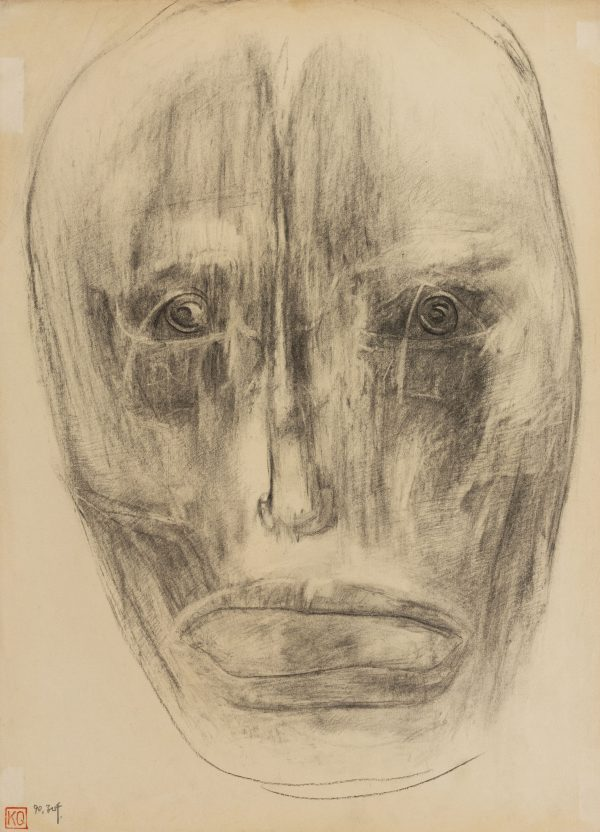1990. 纸本素描. 54.5×39cm.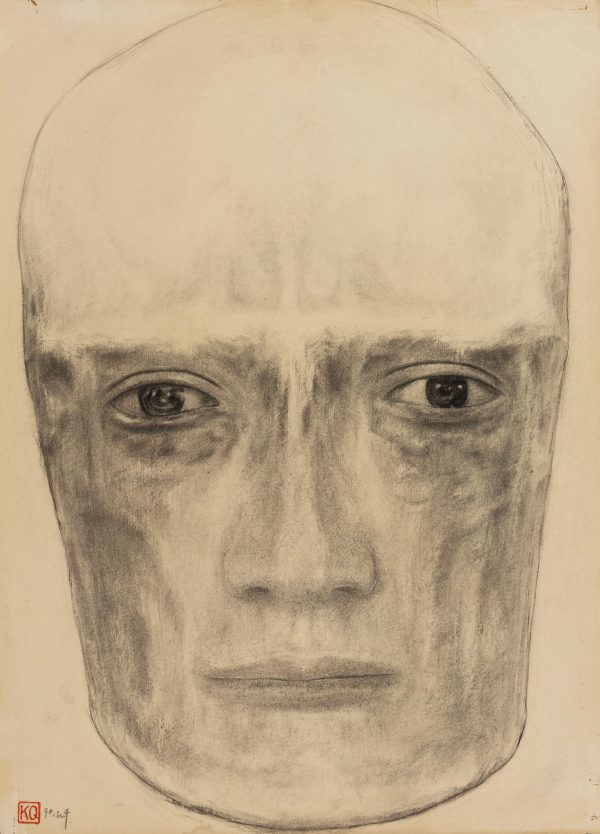1990. 纸本素描. 54.5×39cm.1990. 纸本素描. 54.5×39cm.1990. 纸本素描. 54.5×39cm.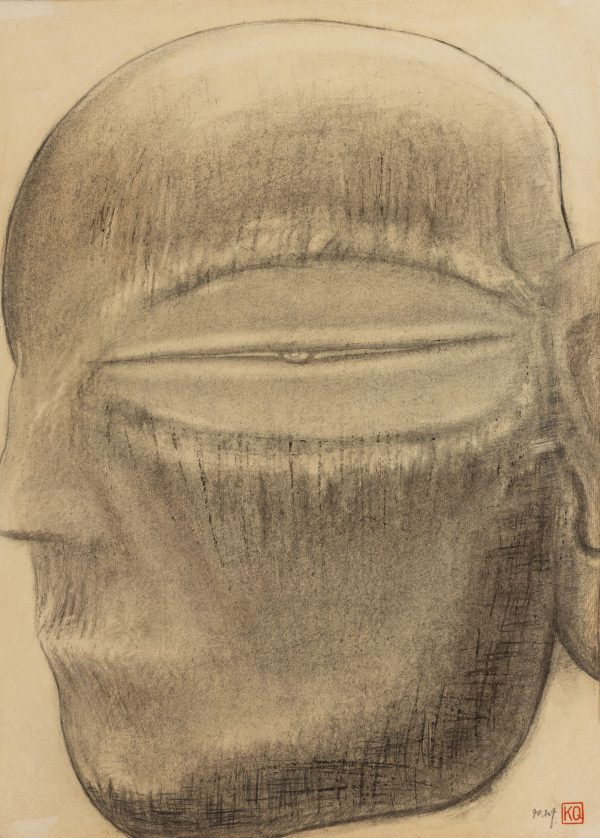1990. 纸本素描. 54.5×39cm.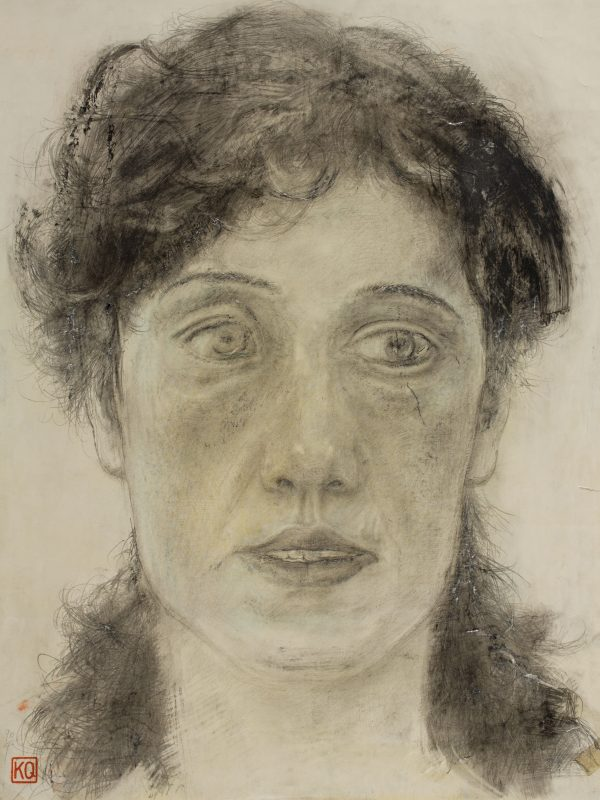1990. 纸本素描. 44×33cm.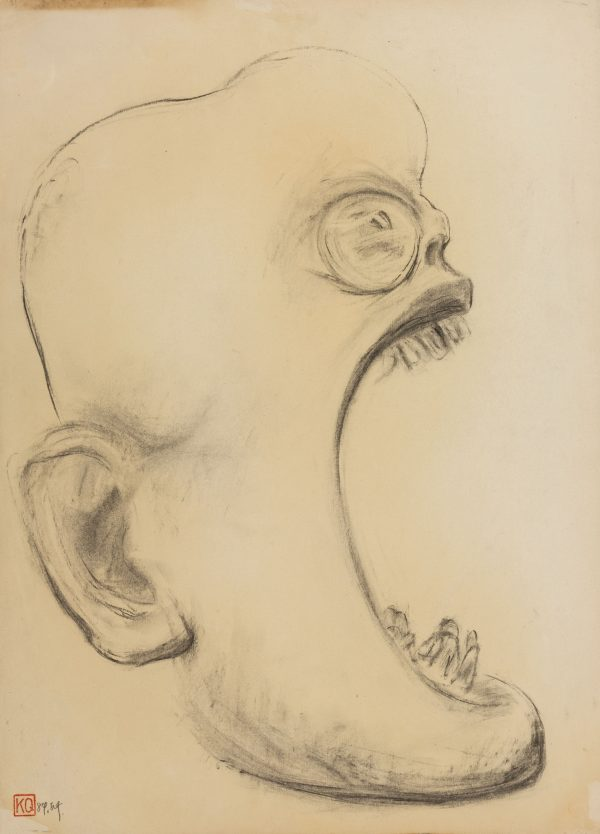1989. 纸本素描. 54.5×39cm.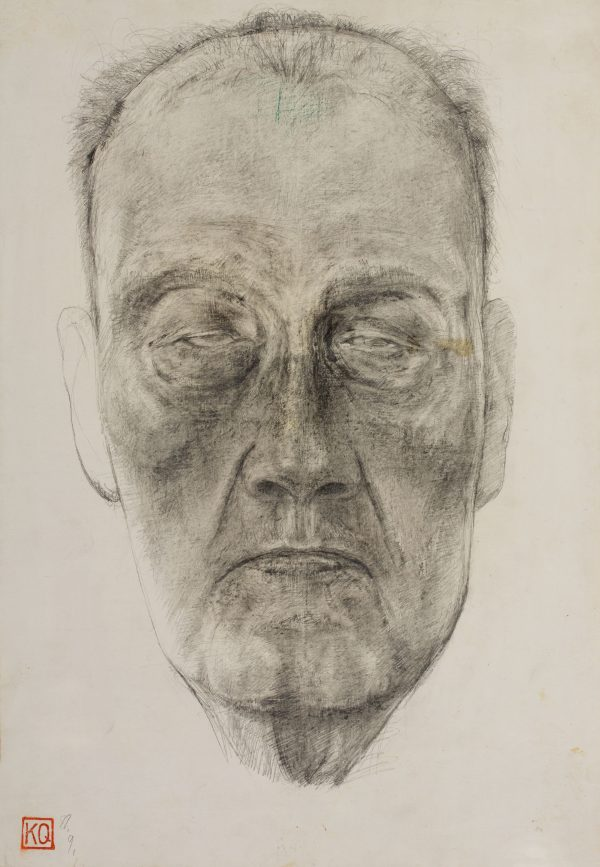1989. 纸本素描. 39.2×27.1cm.1989. 纸本素描. 54.5×39cm.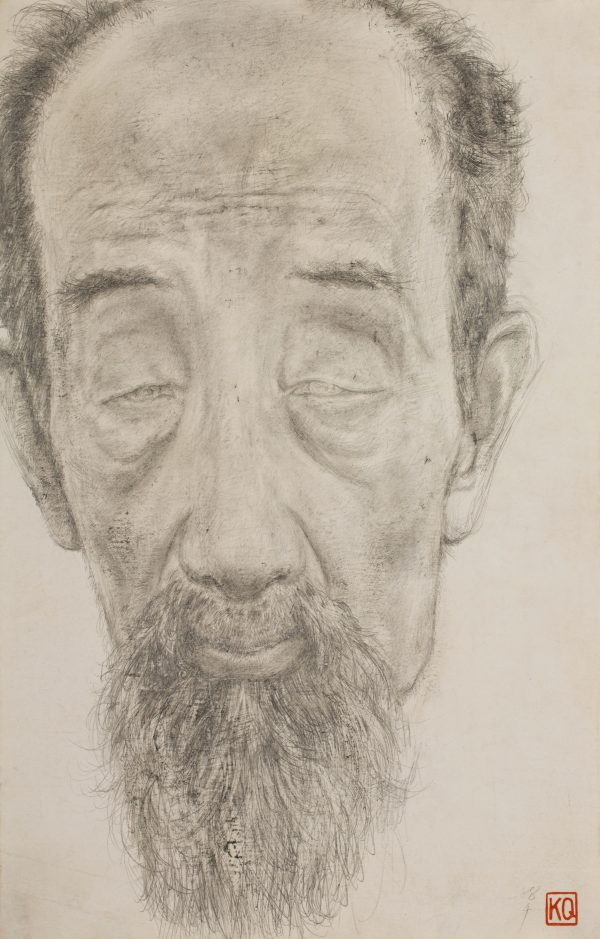1988. 纸本素描. 39.6×27cm.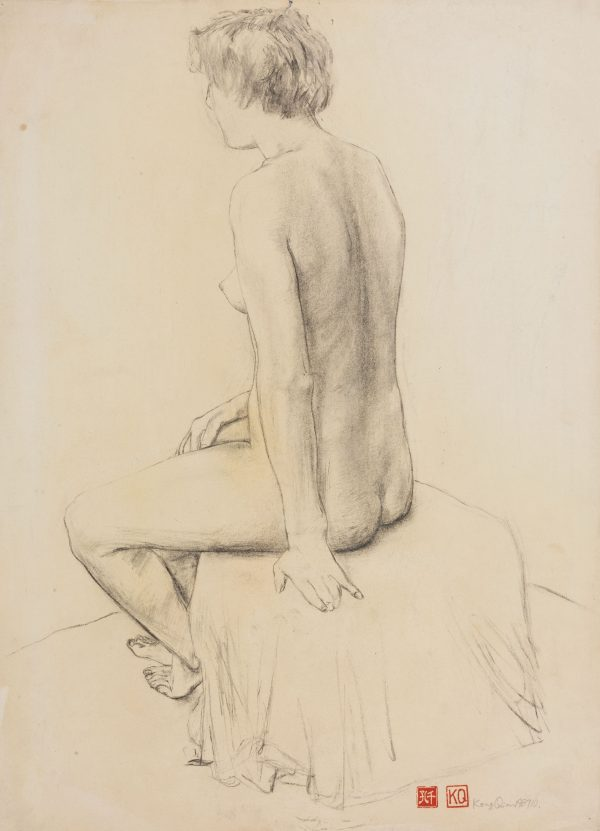1987. 纸本素描. 54.5×39.4cm.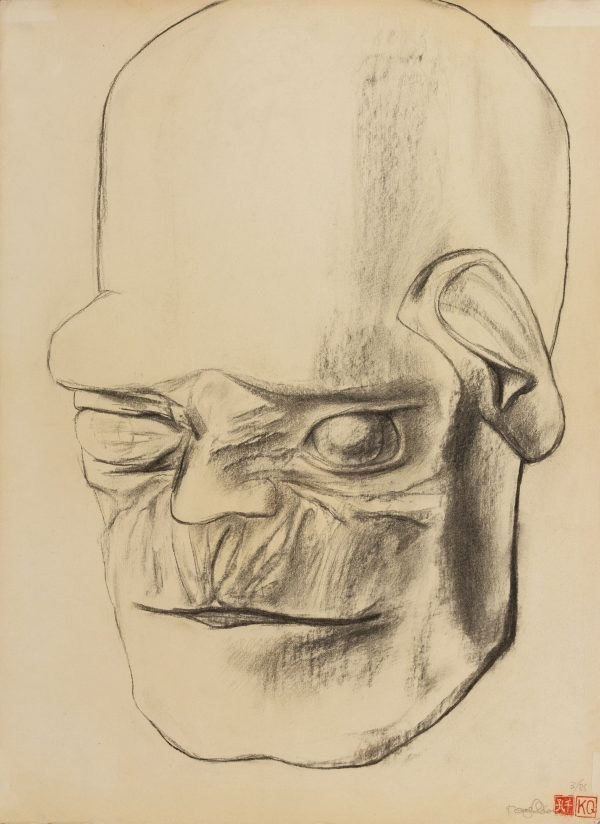1986. 纸本素描. 54.5×39.5cm.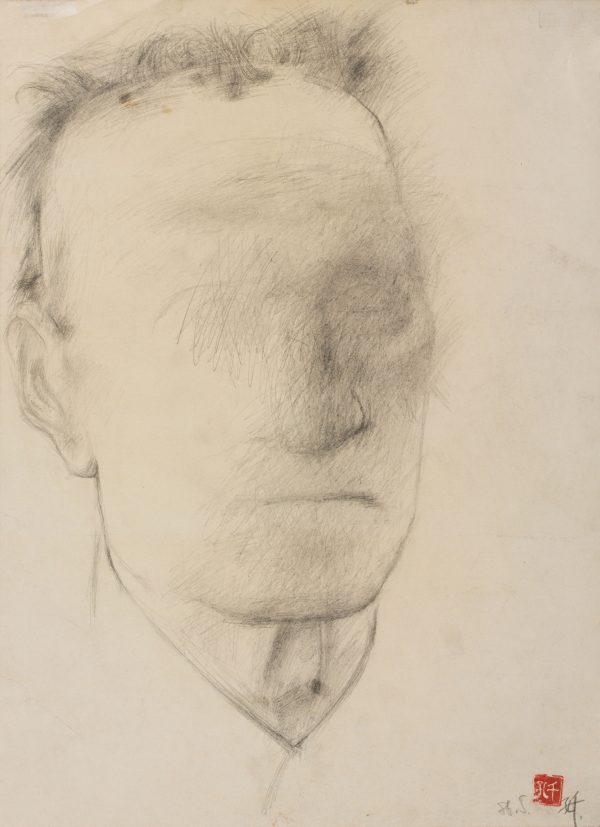1986. 纸本素描. 36.7×26.8cm.

1956年出生于天津的孔千，附中与本科均就读于天津美院。苏派素描作为当时美院的基础教学自然而然成为了青年孔千不断学习与反思的对象。天美毕业之后，孔千在持续对学院艺术价值观进行批判的同时，开始对西方现代主义与中国传统民间艺术广泛吸纳、兼容并蓄，逐渐萌发出其个体化的素描观。自80年代后期开始，素描技艺上的不断锤炼加上外部世界日新月异的变动催化着孔千对于生命意识的体察。孔千进一步通过对具象形象的主观性变形，着力塑造对象的精神状态，在造型上向着愈发奇诡的方向发展，陌生化、异样化的观感在90年代达到顶峰。

2020

## 不合时宜：孔千绘画三十年（1983-2016）

2016